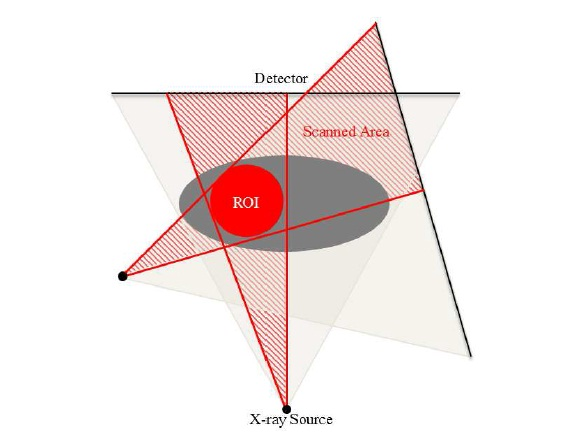Select Page

## Compressed Sensing in Local CTReconstruction

One potential method for reducing the radiation dose is to limit the exposure area to those regions that need to be examined, as illustrated in figure 1. Reconstruction methods based on the FBP technique are non-local because of the use of Fourier transform as its theoretical
foundation. This means that the recovery of any pixel in a CT image requires information from all projections thereby exposing the patient to a relatively large amount of radiation even when only a small region needs to be examined. Several methods for local CT reconstruction have been previously studied. These algorithms are usually based on the localized nature of wavelet transforms , iterative reconstruction
methods , or generalized FBP and truncated Hilbert transform. Here,
we focus on wavelet inverse Radon (WiR) based methods to recover the wavelet subbands of a region of interest (ROI). Another important application of wavelet transform in signal processing is its use as a sparsifying transform in Compressed Sensing. However, since CS-based CT reconstruction methods use the discrete Radon matrix, they are not suitable for local CT reconstruction. In this chapter it is shown that CS-based CT reconstruction and wavelet inverse Radon can be combined to achieve an ultra low dose local CT imaging technique. First, WiR is improved by decreasing its computational load. WiR uses back projection (BP) to recover the wavelet subbands. In the proposed method, denoted by accelerated wavelet inverse Radon
(AWiR), BP is replaced by Direct Fourier Reconstruction (DFR)  on pseudo-polar grids; this significantly reduces the computational complexity. By combining CS with AWiR, we propose a low dose CS-based local CT reconstruction algorithm, denoted by Accelerated Wavelet inverse Radon Subband adaptive Iterative Shrinkage/Thresholding Algorithm (AWiR-SISTA), that takes advantage of both local CT imaging and CS-based CT reconstruction to reconstruct ultra-low dose local CT images. This method, not only reduces the effective radiation, but alsoFigure 1: Region of Interest scanning method.

decreases the computational complexity of CS recovery and, in addition, by using an innovative combination of Two step Iterative Soft Thresholding  and subband dependent CS recovery , the convergence rate of iterative soft thresholding algorithm (ISTA) is improved.

## Wavelet Transform and CT image Reconstruction

The problem of low dose local CT imaging is studied in this chapter by proposing a wavelet subband dependent CS-based CT reconstruction algorithm. To achieve high quality low dose CT images, it should be noted that CS-based CT reconstructions recover the images using fewer projections; and that wavelet inverse Radon algorithms recover wavelet subbands of CT images from locally scanned projections. Moreover, it has been shown that subband CS algorithms accelerate the convergence of the CS recovery methods, such as ISTA (described in Algorithm 1). Here, we propose an innovative combination of a newly developed accelerated wavelet inverse Radon transform and non-convex CS formulation to recover the wavelet subbands of CT images from a reduced number of locally scanned X-ray projections. Fast pseudo-polar Fourier transform is used to accelerate wavelet inverse Radon, and as a result, to decrease the computational complexity of CS recovery. Therefore, the proposed method, denoted by AWiRSISTA, reduces the radiation dose by simultaneously decreasing the X-ray exposure area and the number of projections, decreases the CS computational complexity, and accelerates the CS recovery convergence rate.

https://tspace.library.utoronto.ca/handle/1807/69275